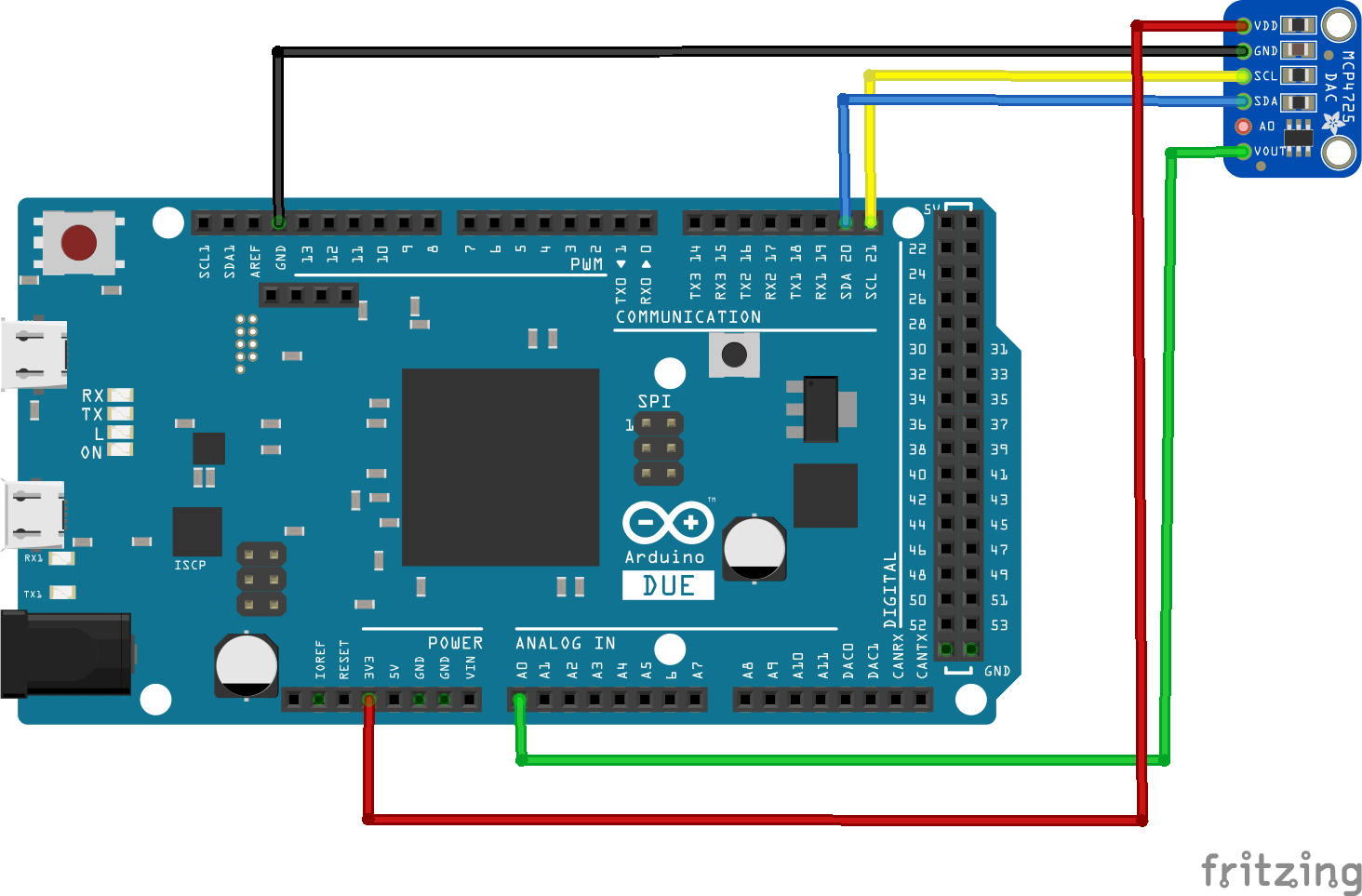Arduino Due and MCP4725 Digital-to-Analog Converter example

MCP4725 is a single channel, 12-bit, voltage output Digital-to-Analog Converter with integrated EEPROM and an I2C Compatible Serial Interface.

Again the easiest way to interface is to purchase a module, these are available from many sources, here is what my one looked like.mcp4725

Features

12-Bit Resolution
On-Board Non-Volatile Memory (EEPROM)
±0.2 LSB DNL (typ)
External A0 Address Pin
Normal or Power-Down Mode
Fast Settling Time of 6µs (typ)
External Voltage Reference (VDD)
Rail-to-Rail Output
Low Power Consumption
Single-Supply Operation: 2.7V to 5.5V
I2CTM Interface:
Standard (100 kbps), Fast (400 kbps) andHigh Speed (3.4 Mbps) Modes
Small 6-lead SOT-23 Package
Extended Temperature Range: -40°C to +125°C

As this is a 12 bit DAC converter. What this means is that it will accept up to 4096 possible inputs to provide an analog output, where an output value of zero is zero and an output value of 4095 is full scale.
Full scale is determined by the reference voltage you supply to the VCC pin. Also you can see from above that the supply voltage can be anywhere from 2.7 volts to 5.5 volts. We will use 5v, or as close as what is supplied via the USB in. You may want to measure this voltage for accurate readings, I’ve seen this vary.

This means that to work out the value of the Least Significant Bit (LSB) is as follows:

1 LSB = VCC Voltage / 4096

Connectionarduino due and mcp4725

Code

This code example uses a modified version of the Adafruit library – Adafruit_MCP4725

Basically we increment the DAC, read in the value and display the results via the serial monitor

 Source code#include <Wire.h>

#define MCP4725In A0

void setup(void)
{
Serial.begin(9600);
MCP4725.begin(0x60); // The I2C Address of my module
}

void loop(void)
{
uint32_t MCP4725_value;
int adcInput = 0;
float voltageIn = 0;

for (MCP4725_value = 0; MCP4725_value < 4096; MCP4725_value = MCP4725_value + 128)
{
MCP4725_reading = (3.3/4096.0) * MCP4725_value; //3.3 is your supply voltage
MCP4725.setVoltage(MCP4725_value, false);
delay(250);
adcInput = analogRead(MCP4725In); //module output connect to A0
voltageIn = (adcInput * 3.3 )/ 1024.0;

Serial.print("\tExpected Voltage: ");

Serial.print("\tArduino Voltage: ");
Serial.println(voltageIn,3);
}
}

Open the serial monitor to see the results